Foxtable(狐表)用户栏目专家坐堂 → 表的汇总并写入问题

共有1685人关注过本帖树形打印复制链接

# 主题：表的汇总并写入问题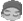skyerlin
1楼 | 信息 | 搜索 | 邮箱 | 主页 | UC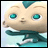加好友发短信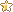以下代码加在“收费明细表”的datacolchanged事件上，要实现当收费明细表有数据变化时，学年收费名单表根据学号和学年自动对收费明细表的各项收费进行求和汇总。Dim dr As  Row=Tables("学年收费名单").CurrentDim sum1,sum2,sum3,sum4 As Integersum1 =  DataTables("收费明细").Compute("sum(实收学费)", "学号='" & dr("学号") & "' And 学年='" & dr("学年") & "'")dr("实收学费") = sum1sum2 =  DataTables("收费明细").Compute("sum(实收住宿费)", "学号='" & dr("学号") & "'And 学年='" & dr("学年") & "'")dr("实收住宿费") = sum2sum3 =  DataTables("收费明细").Compute("sum(实收书本费)", "学号='" & dr("学号") & "'And 学年='" & dr("学年") & "'")dr("实收书本费") = sum3sum4 =  DataTables("收费明细").Compute("sum(实收保险费)", "学号='" & dr("学号") & "'And 学年='" & dr("学年") & "'")dr("实收保险费") = sum4请问代码错在那，如何修改？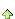2楼 | 信息 | 搜索 | 邮箱 | 主页 | UC加好友发短信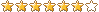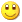Post By：2018/10/11 17:11:00 [只看该作者]

 Dim dr As DataRow = e.DataRowDim fdr As DataRow = DataTables("学年收费名单").find("学号='" & dr("学号") & "' And 学年='" & dr("学年") & "'")If fdr IsNot Nothing Then    Dim sum1,sum2,sum3,sum4 As Integer    sum1 =  DataTables("收费明细").Compute("sum(实收学费)", "学号='" & dr("学号") & "' And 学年='" & dr("学年") & "'")    fdr("实收学费") = sum1    sum2 =  DataTables("收费明细").Compute("sum(实收住宿费)", "学号='" & dr("学号") & "'And 学年='" & dr("学年") & "'")    fdr("实收住宿费") = sum2    sum3 =  DataTables("收费明细").Compute("sum(实收书本费)", "学号='" & dr("学号") & "'And 学年='" & dr("学年") & "'")    fdr("实收书本费") = sum3    sum4 =  DataTables("收费明细").Compute("sum(实收保险费)", "学号='" & dr("学号") & "'And 学年='" & dr("学年") & "'")    fdr("实收保险费") = sum4End Ifskyerlin
3楼 | 信息 | 搜索 | 邮箱 | 主页 | UC加好友发短信Post By：2018/10/11 17:35:00 [只看该作者]

 谢谢。